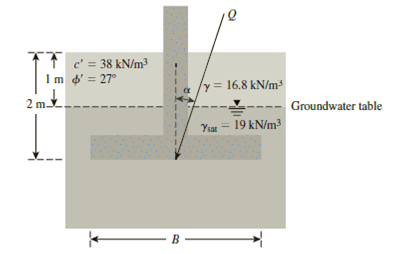Chapter 16, Problem 16.12PPrinciples of Geotechnical Enginee...

9th Edition
Braja M. Das + 1 other
ISBN: 9781305970939

Solutions

Chapter
SectionPrinciples of Geotechnical Enginee...

9th Edition
Braja M. Das + 1 other
ISBN: 9781305970939
Textbook Problem

A square footing is subjected to an inclined load as shown in Figure 16.19. If the size of the footing, B = 2.25 m, determine the gross allowable inclined load, Q, that the footing can safely carry. Given: α = 12° and Fs = 3.5.Figure 16.19

To determine

Find the gross allowable inclined load (Q) that the footing can safely carry.

Explanation

Given information:

The width of footing B is 2.25 m.

The unit weight of the soil γ is 16.8kN/m3.

The saturated unit weight of the soil γsat is 19kN/m3.

The value of cohesion c is 38kN/m2.

The soil friction angle ϕ is 27°.

The depth of foundation Df is 2 m.

The depth of foundation above the water table D is 1 m.

The inclination of the load on the foundation with respect to the vertical α is 12°.

The factor of safety Fs is 3.5.

Calculation:

Determine the shape factor λcs using the relation.

λcs=1+(BL)(NqNc)

Here, Nq is the contribution of surcharge, Nc is the contribution of cohesion,

Refer Table 16.2, “Bearing-capacity factors Nc, Nq, and Nγ” in the textbook.

Take the Nc as 23.94, Nq as 13.20, and Nγ as 14.47 for the ϕ value of 27°.

Substitute 2.25 m for B, 2.25 m for L, 13.20 for Nq, and 23.94 for Nc.

λcs=1+(2.252.25)(13.2023.94)=1.55

Determine the depth factor λcd using the relation.

λcd=1+0.4(DfB)

Substitute 2 m for Df and 2.25 m for B.

λcd=1+0.4(22.25)=1.355

Determine the inclination factor λci using the relation.

λci=λqi=(1α90°)2

Substitute 12° for α.

λci=(112°90°)2=0.751

Determine the shape factor λqs using the relation.

λqs=1+(BL)tanϕ

Substitute 2.25 m for B, 2.25 m for L, and 27° for ϕ.

λqs=1+(2.252.25)tan27°=1.509

Determine the depth factor λqd using the relation.

λqd=1+2tanϕ(1sinϕ)2(DfB)

Substitute 27° for ϕ, 2 m for Df, and 2.25 m for B.

λqd=1+2tan27°(1sin27°)2(22.25)=1.27

Determine the shape factor λγs using the relation.

λγs=10.4(BL)

Substitute 2.25 m for B and 2.25 m for L.

λγs=10.4(2.252.25)=0.6

Determine the inclination factor λγi using the relation.

λγi=(1αϕ)2

Substitute 12° for α and 27° for ϕ.

λγi=(112°27°)2=0.308

Determine the magnitude of q using the relation.

q=γ(DfD)+γD=γ(DfD)+(γsatγw)D (1)

Here, γ is the effective unit weight of soil and γw is the unit weight of water

Still sussing out bartleby?

Check out a sample textbook solution.

See a sample solution

The Solution to Your Study Problems

Bartleby provides explanations to thousands of textbook problems written by our experts, many with advanced degrees!

Get Started

Find more solutions based on key concepts